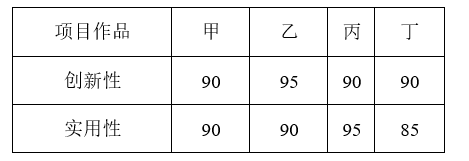$\text{A.}$ 甲 $\text{B.}$ 乙 $\text{C.}$ 丙 $\text{D.}$ 丁